Chapter 5: Trusses

# 5.5 Examples

Here are examples from Chapter 5 to help you understand these concepts better. These were taken from the real world and supplied by FSDE students in Summer 2021. If you’d like to submit your own examples, please send them to the author eosgood@upei.ca.

# Example 5.5.1: Method of Sections – Submitted by Riley Fitzpatrick

1. Problem

A flower cart at a local garden center is being pushed with a force of 500 n at joint G. It’s back wheels (A) are locked so it is not moving. There is 1 meter of space between each of the four shelves in height and each shelf is four meters long.

a) Calculate the reaction forces of the locked wheels and the unlocked wheels.

b) Calculate the load carried by FCG, and whether it is in tension or compression

Which method did you use, joints or sections? Which is faster for the style of questions? How would your strategy change if your were calculating the load in each member?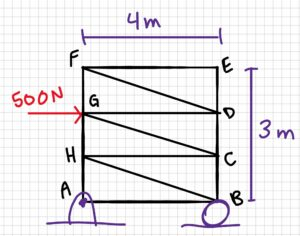2. Draw

Sketch:Free-body Diagram: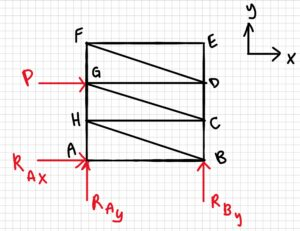3. Knowns and Unknowns

Known:

• P = 500 N
• Width = 4 m
• Total height = 3 m

Unknowns: RAx, RAy, RBy, FCG

4. Approach

Part a: determine reaction forces using equilibrium equations

Part b: calculate FCG using method of sections. Make a cut, then solve internal forces using equilibrium equations

5. Analysis

Part a:

Solving for RAx:

$$\sum F_x=0=P-R_{Ax}\\R_{Ax}=P\\R_{Ax}=500N$$

Solving for RBy:

$$\sum M_A=0=(r_{BA}\cdot R_{By})-(r_{GA}\cdot P)\\r_{BA}\cdot R_{By}=r_{GA}\cdot P\\R_{By}=\frac{r_{GA}\cdot P}{r_{BA}}\\R_{By}=\frac{2m\cdot 500N}{4m}\\R_{By}=250N$$

Solving for RAy:

$$\sum F_y=0=R_{By}+R_{Ay}\\R_{Ay}=-R_{By}\\R_{Ay}=-250N$$

The answer we got is a negative number. All this means is that the direction of this vector is drawn wrong on our original diagram (in reference to our coordinate frame). This makes sense as RAy and RBy are the only external forces in the y direction, so they have to cancel each other for the equilibrium equations to be true. Therefore, one of them should have a negative direction. We will leave this answer as is for now, but the next time we draw the system, we will change the direction of the arrow.

$$\underline{R_{Ax}=500N,\; R_{Ay}=-250N, \; R_{By}=250N}$$

Part b:

Firstly, we re-draw the diagram, changing the direction of RAy. Then, since we are using method of sections, we make a cut so that the member FCG (the one we want to find) is cut.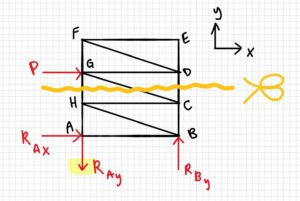Now we re-draw, choosing one of the pieces from the cut. Here the top half is chosen, but you could also chose the bottom half and get the right answer. The only reason the top half was chosen here is because there are less external forces to consider for the top.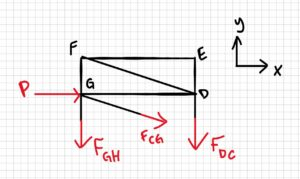Solve for the member we are looking for:

$$\sum F_x=0=P+\frac{4}{\sqrt{17}}F_{CG}\\\frac{4}{\sqrt{17}}F_{CG}=-P\\F_{CG}=-P(\frac{\sqrt{17}}{4})\\F_{CG}=-500N(\frac{\sqrt{17}}{4})\\F_{CG}=-515.388N$$

Again, the number we get is negative. The way we drew FCG originally was as if the member was in tension. The negative number just means that it is actually compression, not tension.

$$\underline{F_{CG}=515 \text{N (Compression)}}$$

Part c:

For part b I used the method of sections, as it would be the fastest method. The method of joints would require the lower joints to be solved first which would be a much slower process, whereas with this method a simple cut can be made and the member;s load can be quickly solved using equilibrium equations. Had the question asked for all member loads to be solved however, the method of joints would have been the faster approach.

6. Review

Part a:

RAx is equal and opposite to P so we know it is correct, and the value of RBy should also be correct as its moment about A (250 * 4m = 1000 Nm), is equal and opposite to the moment of P about A, (500 N * 2 m = 1000 Nm). as RAy is equal and opposite to RBy it is also correct.

Part b:

The x component of the calculated value of FCG is equal in magnitude to P (see equation below), and it is the only cut member acting in the x direction. Therefore, it must be correct.

$$\frac{4}{\sqrt{17}}(515 N)=500 N$$

Part c:

The method of sections allows you to solve a very specific area of the systems internal forces (the members that are cut), whereas the method of joints usually requires you to solve most if not all of the internal forces of the system. Therefore, the method of sections is the most efficient for finding the internal forces of specific parts of the system, whereas the method of joints is more efficient for solving the whole system.

# Example 5.5.2: Zero-Force Members, submitted by Michael Oppong-Ampomah

1. Problem

A bridge with uneven ground has been built as shown below. Force is applied at three points on the top of the bridge.

a). Find any zero-force members

b). What purpose do these members serve?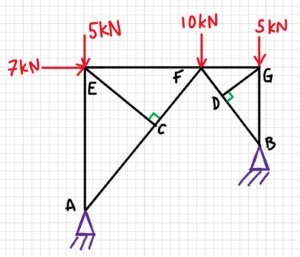2. Draw

Free-body diagram: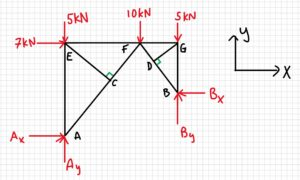3. Knowns and Unknowns

Unknown: which members are zero-force

4. Approach

Look at each joint and determine how many forces are in each direction. If there is only one force in a direction, that member is zero-force.

5. Analysis

Part a:

Let’s start with joint C. If we think of the forces acting in the x and y directions as shown below by the coordinate frame, we see that there are two forces acting in the y direction, and only one in the x direction. Therefore, assuming the joint is in static equilibrium, member CE is zero-force.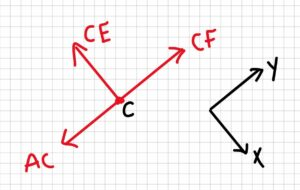If we do the same type of analysis for the other joints and remove the zero-force members, the structure now looks like this: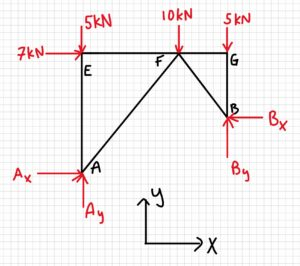After one more analysis of the joints, we find one more zero-force member, as shown below.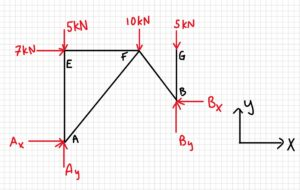Answer: CE, DG, and FG are zero-force members.

Part b:

Zero-force members exist to provide stability to the truss, to keep the shape rigid.

6. Review

Although the new truss (without zero-force members) looks strange, there are no joints where there’s only one force in one direction, therefore there are no more zero-force members.